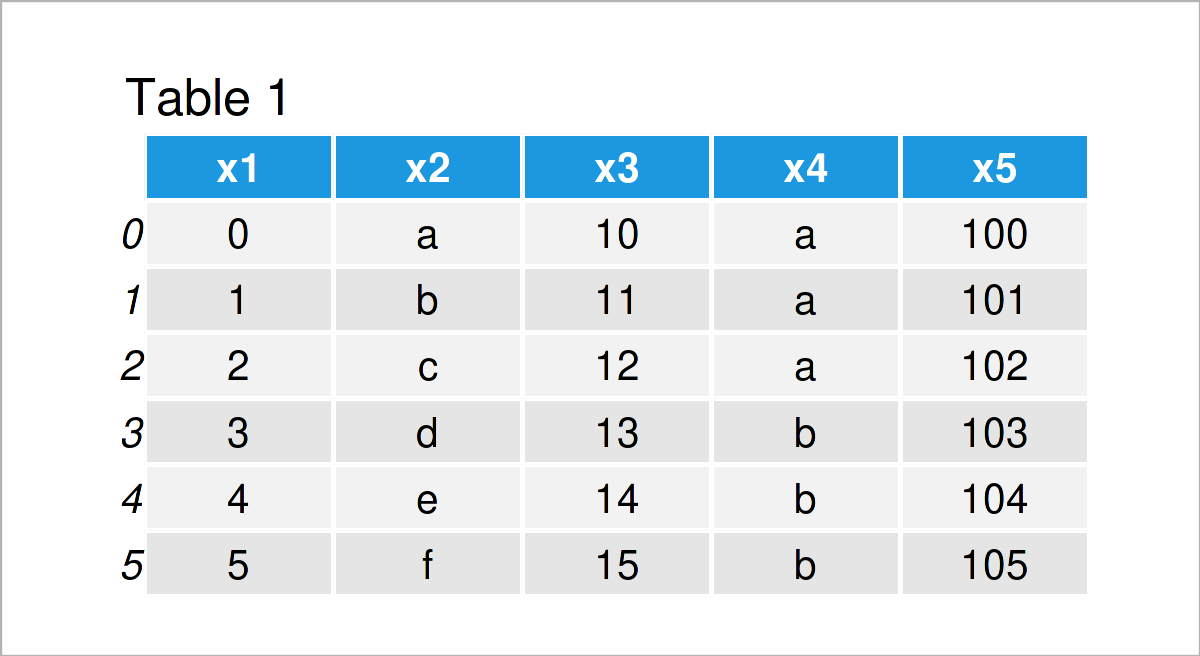# Compare Headers of Two pandas DataFrames in Python (3 Examples)

In this tutorial, I’ll demonstrate how to compare the headers of two pandas DataFrames in Python.

Let’s dive right into the examples:

## Example Data & Libraries

We first have to import the pandas library:

`import pandas as pd                               # Import pandas library`

Furthermore, consider the following two example DataFrames:

```data1 = pd.DataFrame({'x1':range(0, 6),           # Create first pandas DataFrame
'x2':['a', 'b', 'c', 'd', 'e', 'f'],
'x3':range(10, 16),
'x4':['a', 'a', 'a', 'b', 'b', 'b'],
'x5':range(100, 106)})
print(data1)                                      # Print first pandas DataFrame``````data2 = pd.DataFrame({'x1':range(25, 30),         # Create second pandas DataFrame
'x2':['x', 'y', 'z', 'z', 'x'],
'x5':range(30, 25, - 1),
'x6':range(50, 45, - 1)})
print(data2)                                      # Print second pandas DataFrame```Our two example pandas DataFrames are shown in Tables 1 and 2. As you can see, both DataFrames do partly have the same column names, but some of the columns are only contained in one of the data sets.

Let’s inspect these similarities and differences systematically!

## Example 1: Find Columns Contained in Both pandas DataFrames

In this example, I’ll demonstrate how to identify all columns that are contained in both pandas DataFrames.

For this task, we can apply the intersection function as shown below:

```print(data1.columns.intersection(data2.columns))  # Apply intersection function
# Index(['x1', 'x2', 'x5'], dtype='object')```

Have a look at the previous output of the Python console: It shows the column names x1, x2, and x5, i.e. the variables that both pandas DataFrames have in common.

## Example 2: Find Columns Only Contained in the First pandas DataFrame

In this example, I’ll demonstrate how to find columns that are only contained in the header of the first input DataFrame.

To accomplish this, we can use the difference function as shown below:

```print(data1.columns.difference(data2.columns))    # Apply difference function
# Index(['x3', 'x4'], dtype='object')```

As you can see based on the previous output, the variables x3 and x4 do only exist in the first data set.

## Example 3: Find Columns Only Contained in the Second pandas DataFrame

Similar to Example 2, we can also investigate the column names that are only contained in the second data set.

To achieve this, we simply have to revert the order of the two data sets when applying the difference function:

```print(data2.columns.difference(data1.columns))    # Apply difference function
# Index(['x6'], dtype='object')```

The column x6 is only contained in the second pandas DataFrame.

## Video & Further Resources

Do you need further explanations on the contents of this tutorial? Then you may want to have a look at the following video on my YouTube channel. In the video, I illustrate the Python code of this tutorial in the Python programming language.

Furthermore, you may want to have a look at the related Python tutorials on my website. I have released numerous articles about similar topics such as merging and indices.

Summary: In this tutorial, I have explained how to compare and find differences between the headers of two pandas DataFrames in the Python programming language. If you have any further questions, let me know in the comments section.

Subscribe to the Statistics Globe Newsletter